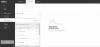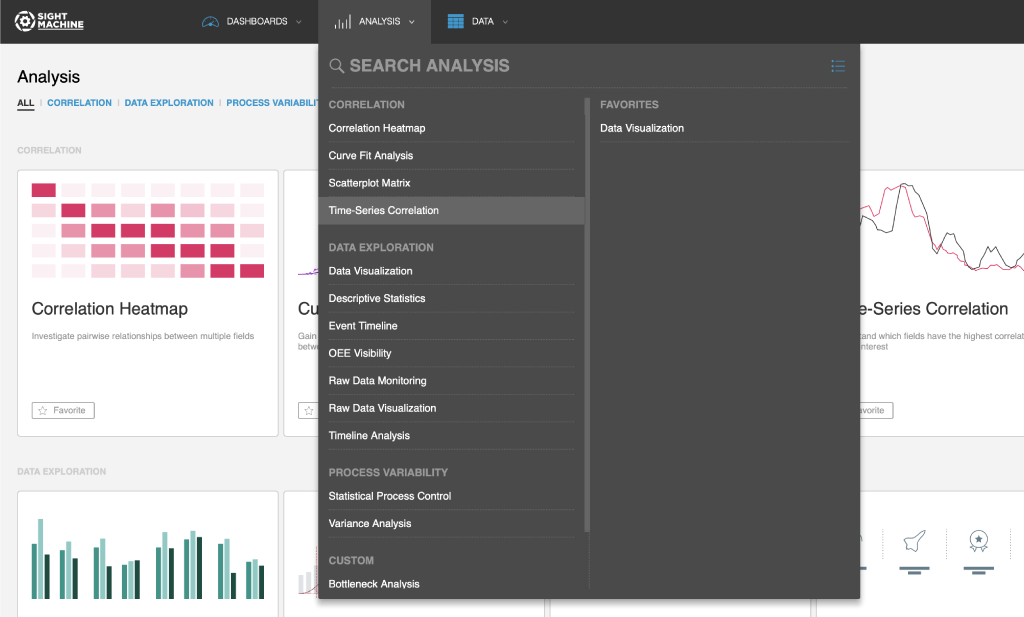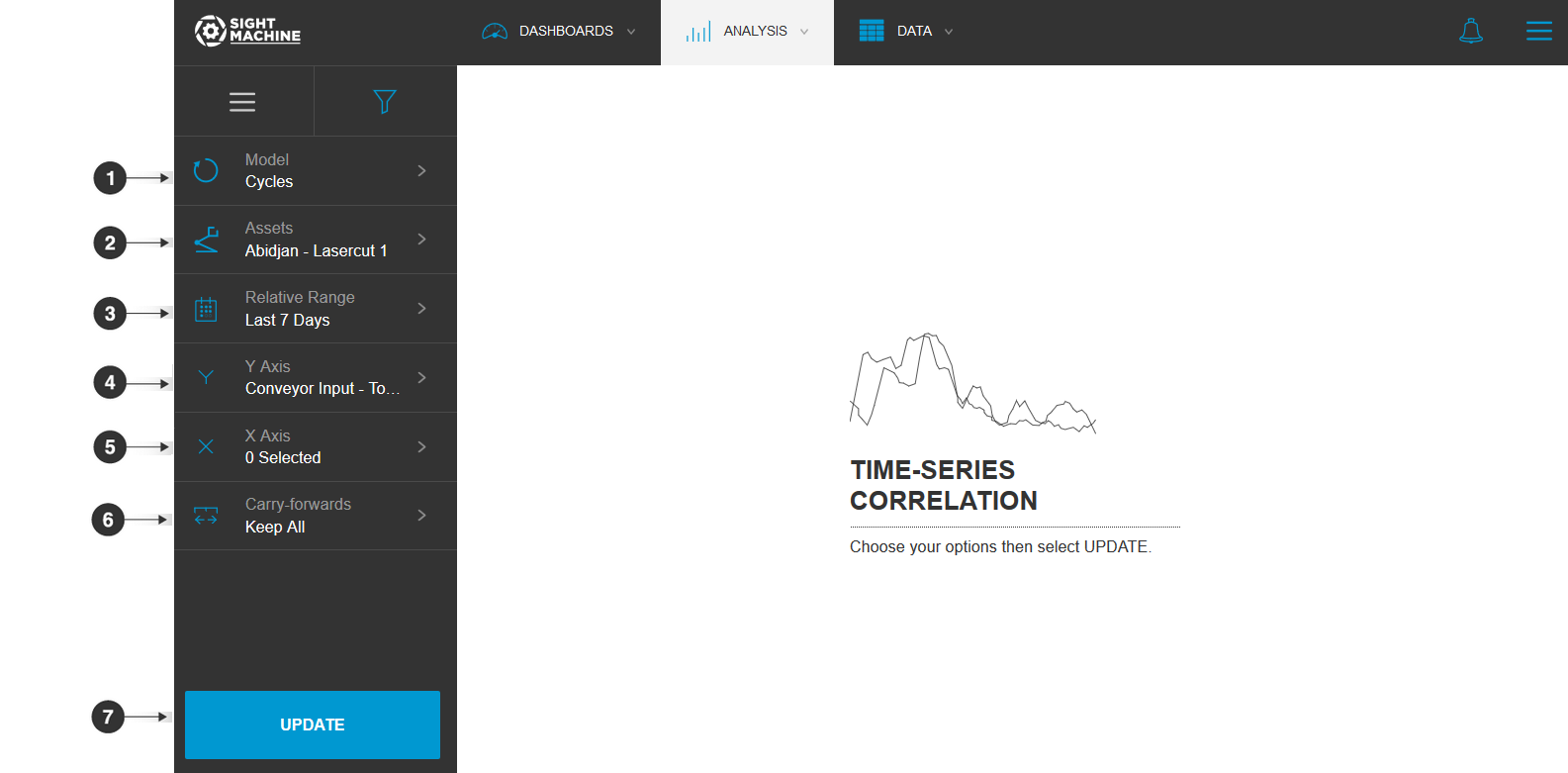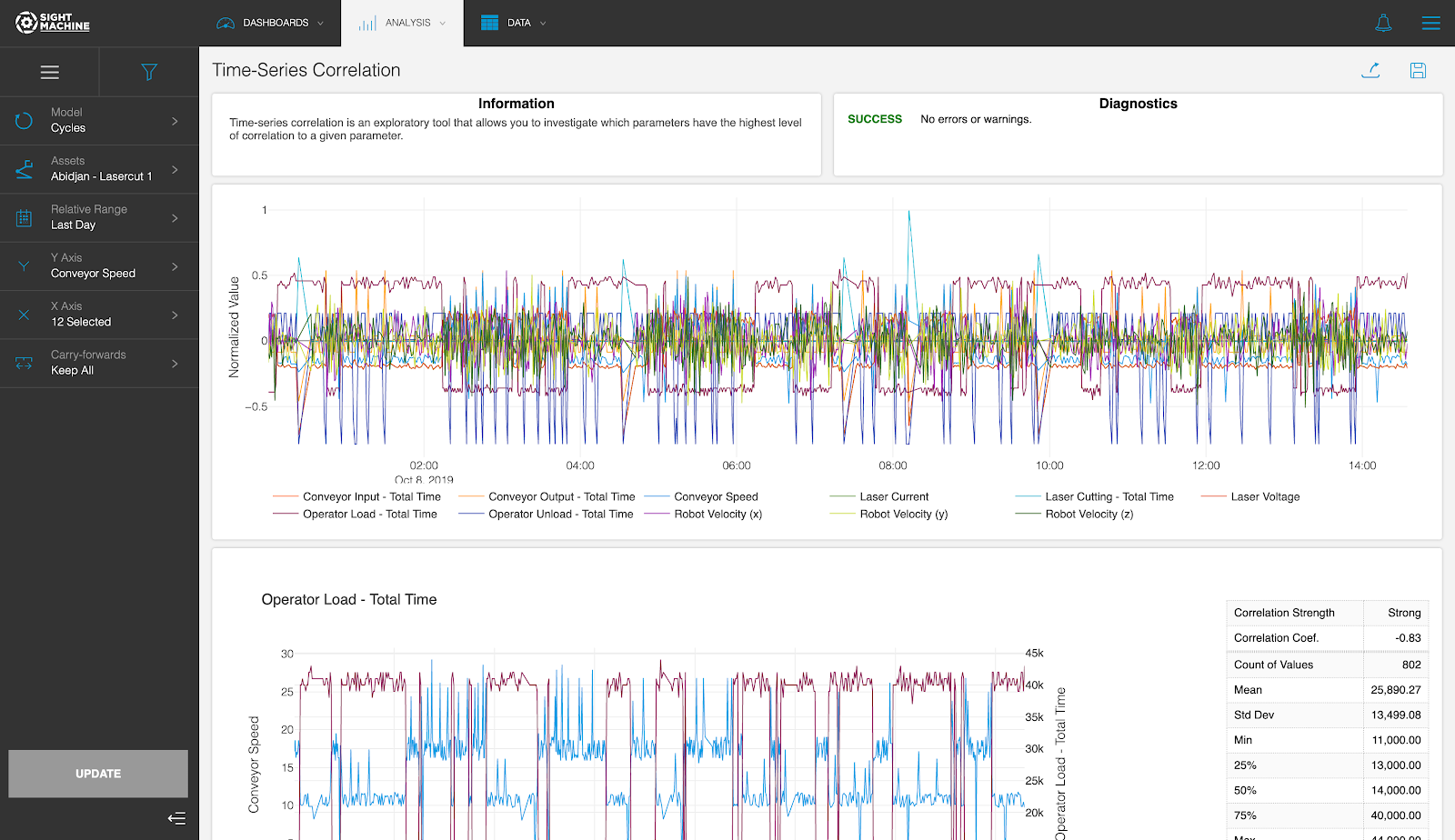# Time-Series Correlation# Use Cases

The Time-Series Correlation discovers parameters that have high correlation with the selected parameter, taking the burden of discovery from you (compared to tools like the Correlation Heatmap, which requires active parameter selection). This tool compares the selected parameter with all parameters except those that you excluded, ranks the pairs by the magnitude of their correlation coefficients, and provides details about the top 10 pairs. The Time-Series Correlation is most helpful early in the exploratory process to surface high correlations that you want to investigate in more detail. This is a good tool to lead to Curve Fit Analysis.

# Specifications

The Time-Series Correlation is a multivariate analysis that you can perform on continuous fields on the Cycle and Part models. Using the Cycle model, the Time-Series Correlation can investigate parameters from the same machine type with high granularity. Using the Part model lets you compare fields across different machines, but with lower granularity (the Part model is limited by the serial number or other identifier used to construct the data model).

# Analysis

The Time-Series Correlation allows you to select one parameter of interest and any number of other parameters to analyze. The Time-Series Correlation computes the Pearson-R correlation coefficient between the selected parameter and each of the others. The first plot shows all parameters over time for the selected date range. For each of the top 10 pairs (ranked by correlation coefficient), the tool plots the two parameters over time and presents summary statistics including Count, Standard Deviation, Minimum, and Maximum.

# How to Use a Time-Series Correlation

The Time-Series Correlation tool allows you to investigate which parameters have the highest level of correlation to a given parameter.

NOTE: Correlation is limited to continuous/numeric parameters.

To use a Time-Series Correlation:

1. On the Analysis tab, under Correlation, click Time-Series Correlation.2. On the main Time-Series Correlation screen, select your options on the left. For more details about each option, see Time-Series Correlation Options.
3. Click Update.

# Time-Series Correlation OptionsThe Time-Series Correlation options include:

1. Model: You can select:
• Cycles: Examine the correlation between two parameters in the same asset or asset type.
• Parts: Examine the correlation between two parameters across different machines producing the same part type.
2. Assets: You can select assets to monitor. For example, to perform a Cycles analysis, you need to select an asset (or multiple assets of same type) to analyze. You can select 2 specific machines. If you are using the Parts model, you need to select a part type.
3. Relative Range: You can define a date range of data points to analyze for the assets you have selected. For example, select the last 7 days. This option establishes the boundaries for the near real-time data that will be part of your analysis. Select from relative or absolute timeframes.
4. Y Axis: You can select the parameter that you want to analyze to be plotted along the Y axis.
5. X Axis: You can select a second parameter that you want to analyze to be plotted along the X axis.
6. Carry-forwards: You can undo the forward-fill effect on any fields. Select from Keep All, First, or Last. For more information, see the table in Options Common to All Analysis Tools.
7. Update: Click this button to generate your chart.# Tool Output

The Time-Series Correlation tool computes the correlation between the selected parameter (displayed on the Y axis) and all other continuous/numeric parameters. It then displays the top 10 parameters that have the highest correlation coefficient and produces 11 time series charts, as follows:

• The first chart displays all 11 parameters (the selected parameter and the top 10 most correlated parameters).
• Below that are 10 additional time series plots, one for each parameter. These visualizations and associated shapes allow you to gain a deeper understanding of the relationship between two parameters.